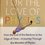# Testing paint skills( physics question)

Hi , i made this question yesterday, and it follows :

Consider an infinitely large wire (blue) having charge per unit length $$+ \lambda$$, and a finite length wire (red) of length $$l$$ and mass $$m$$ having the same charge per unit length. Its lower end rests at a height of $$h$$ units from the blue wire,as shown in the figure.Image text

Your task is simple. Find the frequency of oscillation of red wire on being disturbed by a small distance vertically6 years, 8 months ago

This discussion board is a place to discuss our Daily Challenges and the math and science related to those challenges. Explanations are more than just a solution — they should explain the steps and thinking strategies that you used to obtain the solution. Comments should further the discussion of math and science.

When posting on Brilliant:

• Use the emojis to react to an explanation, whether you're congratulating a job well done , or just really confused .
• Ask specific questions about the challenge or the steps in somebody's explanation. Well-posed questions can add a lot to the discussion, but posting "I don't understand!" doesn't help anyone.
• Try to contribute something new to the discussion, whether it is an extension, generalization or other idea related to the challenge.

MarkdownAppears as
*italics* or _italics_ italics
**bold** or __bold__ bold
- bulleted- list
• bulleted
• list
1. numbered2. list
1. numbered
2. list
Note: you must add a full line of space before and after lists for them to show up correctly
paragraph 1paragraph 2

paragraph 1

paragraph 2

[example link](https://brilliant.org)example link
> This is a quote
This is a quote
    # I indented these lines
# 4 spaces, and now they show
# up as a code block.

print "hello world"
# I indented these lines
# 4 spaces, and now they show
# up as a code block.

print "hello world"
MathAppears as
Remember to wrap math in $$ ... $$ or $ ... $ to ensure proper formatting.
2 \times 3 $2 \times 3$
2^{34} $2^{34}$
a_{i-1} $a_{i-1}$
\frac{2}{3} $\frac{2}{3}$
\sqrt{2} $\sqrt{2}$
\sum_{i=1}^3 $\sum_{i=1}^3$
\sin \theta $\sin \theta$
\boxed{123} $\boxed{123}$

Sort by:

Hi Jatin!

Here's my solution:

At equilibrium,

$\displaystyle mg=\frac{\lambda^2}{2\pi \epsilon_0}\ln\left(\frac{h+l}{h}\right)$

Lets displace the red wire vertically upwards by $y$.

The net force acting on the red wire is:

$\displaystyle F_{net}=\frac{\lambda^2}{2\pi\epsilon_0}\ln\left(1+\frac{l}{h+y}\right)-mg$

Using the approximation,

$\displaystyle \ln\left(1+\frac{l}{h+y}\right)\approx \ln\left(\frac{h+l}{h}\right)-\frac{ly}{h(l+h)}$

$\displaystyle F_{net}=-\frac{\lambda^2ly}{2\pi h(l+h)\epsilon_0} \Rightarrow \ddot{y}=-\frac{\lambda^2l}{2m\pi h(l+h)\epsilon_0}y$

The above equation is for SHM and the frequency can be easily deduced.

Is this correct?

I have a small question, how do you take such approximations? I had to use Wolfram Alpha, can you provide some help on this? Many thanks! :)

- 6 years, 8 months ago

It was correct!, but you missed $m$ in the denominator, i do these approximations as:

$d(\ln (1 +\frac{l}{h})) = \frac{1}{1 + \frac{l}{h}} \times \frac{- l}{h^2} dh$, where $dh = y$, $d$ representing small change.

- 6 years, 8 months ago

Thanks Jatin! Yes, I missed the m, sorry about that, I will edit that. :)

I was wondering if you don't mind, could you please repost the same physics problem about force on mirror you posted before, I am very interested to know about its solution. Thanks! :)

- 6 years, 8 months ago

It was something where we had to apply gauss law in mirror problem, but since, i was a beginner that time in making problems i later noted that it had some problem, So, i had to delete it to avoid confusion, but still i can at least e-mail it at your id, which you might tell.

- 6 years, 8 months ago

Ah, I don't like to post my address in public but I remember you posted your e-mail id in one of the discussions. I remember your e-mail address so I will send you a mail. :)

- 6 years, 8 months ago

You should also have shown how force of interaction is $\frac{\lambda^2}{2 \pi \epsilon_{0}} \ln\bigg(\frac{h + l}{h}\bigg)$, I do it here,

Consider a small element of length $dx$ having charge $d q = \lambda dx$ at a distance $x$ from blue wire, we know that

$E_{x} = \frac{\lambda}{2 \pi \epsilon_{0} x}$

$F = \displaystyle \int_{h}^{h+l} E_{x} \lambda dx = \int_{h}^{h+l} \frac{\lambda}{2 \pi \epsilon_{0} x} \lambda dx$

= $\frac{\lambda^2}{2 \pi \epsilon_{0}} \ln\bigg(\frac{h + l}{h}\bigg)$

- 6 years, 8 months ago

How did you approximate it ??

- 6 years, 8 months ago

lambda/root(8pie^2epsilon zero*(h+l))...I think this is the answer..if solution is needed I will provide one later..a lil' bit busy!

- 6 years, 8 months ago

No, this is not the answer.

- 6 years, 8 months ago

may have done something wrong during the approximation..sorry!

- 6 years, 8 months ago

Challenge

Solve this within a minute:

We remove the blue wire and and take the red wire to a place where, electric field varies as $E =E_{0} e^{-x^2}$ It stays in equilibrium at some height $H$ say,and i repeat the same experiment, Task remains same , find the frequency of oscillations.

- 6 years, 8 months ago

S I unit of force

- 6 years, 3 months ago